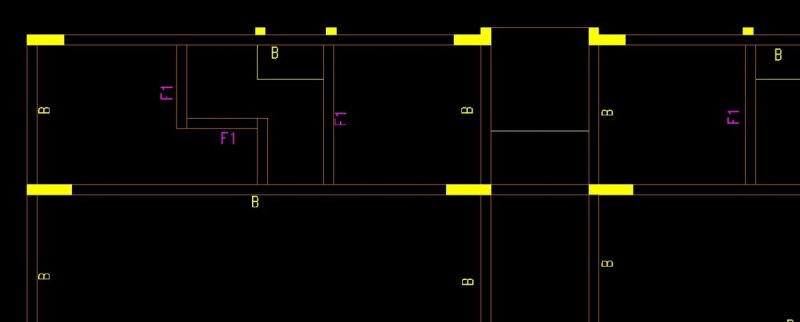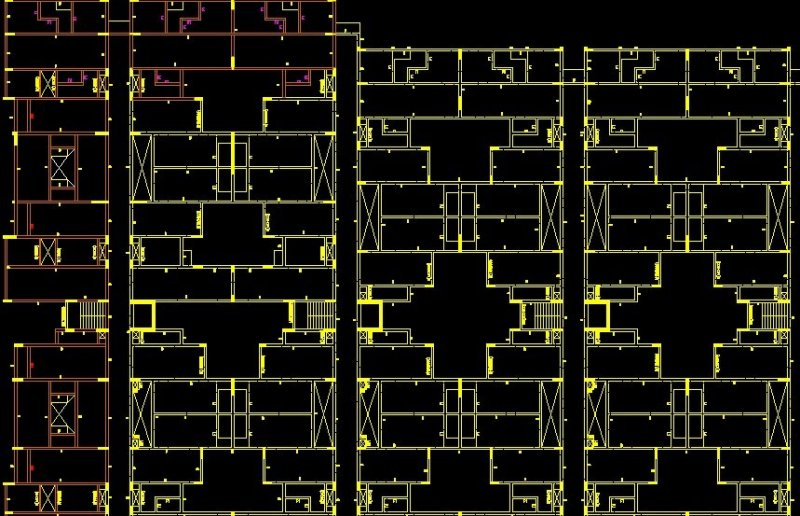length and breadth of rectangles to excel

Recommended PostsDear Forum.

I have over 1000's of rectangles which represent walls.

i need to extract length and breadth of the rectangles while applying a unique naming code to each rectangle and want the results to be tabulated in excel format

RG1                                1500                      150

RG2                                  850                      150

and so on.....Share on other sitesWhen dealing with plines your rectangs, you can simply get the vertices then get the distance between pt1-pt2 and pt2 - pt3 ie length & breadth. Ignore short- long for moment.

The simplest then is to export a csv. You can do direct to excel but adds a extra level of complexity.

The other option is to label each rectang. Again in some sort of order an extra level of complexity.

What is your skill with lisp ? This is the vertice part the points are in the list co-ordsxy

By the way if your rectangs are lines no way to do much.

pline co-ords2.lsp

Share on other sitesShare on other sitesronjonp did you solve this problem before ? Pretty sure its been done just takes some time to find.

Share on other sites12 minutes ago, BIGAL said:

ronjonp did you solve this problem before ? Pretty sure its been done just takes some time to find.

Probably .. but I hate guessing. It could be very simple or very complex based on how the drawing is put together. Too many times code is written to decipher a poorly drafted plan.Share on other sitesAgree this is a simple test pick only a few rectangs.

plinelen-width.lsp

Share on other sites5 hours ago, ronjonp said:

HI ronjonp.

PFA drawing file they are not actually wall but rectangles which i have drafted over the wall layout.

i need to extract length and breadth of the rectangles while naming them uniquely and exported to excel.

Thanking you.

RECTANGLE LANDB.dwg

Share on other sites6 hours ago, BIGAL said:

What is your skill with lisp

hi BIGAL.

My skill with LISP is at basic level not enough to stitching code as per your provided directions.

i had started referring lessons and learning lisp from.

Share on other sites```(defun c:foo (/ a b c d e l n p pr s)
(defun _wf (fn l / f)
(cond ((and (eq 'str (type fn)) (setq f (open fn "w")))
(foreach x l (write-line x f))
(close f)
fn
)
)
)
(setq pr "RG")
(cond	((and (setq s (ssget "_a" (list '(0 . "lwpolyline") '(90 . 4) (cons 410 (getvar 'ctab))))))
(setq l '("ID,LENGTH,WIDTH"))
(setq n 0)
(foreach x (vl-remove-if 'listp (mapcar 'cadr (ssnamex s)))
(vla-getboundingbox (vlax-ename->vla-object x) 'a 'b)
(setq c (mapcar 'vlax-safearray->list (list a b)))
(setq d (vl-sort (mapcar 'abs (mapcar '- (car c) (cadr c))) '>))
(setq p (mapcar '+ (mapcar '/ (mapcar '+ (car c) (cadr c)) '(2. 2.))))
(entmakex (list '(0 . "TEXT")
'(100 . "AcDbEntity")
'(8 . "ID_Text")
'(100 . "AcDbText")
(cons 10 p)
(cons 1 (setq e (strcat pr (itoa (setq n (1+ n))))))
'(50 . 0.0)
'(41 . 1.0)
'(51 . 0.0)
'(7 . "Standard")
'(71 . 0)
'(72 . 1)
(cons 11 p)
'(210 0.0 0.0 1.0)
'(100 . "AcDbText")
'(73 . 2)
)
)
(setq
l (cons (strcat e "," (vl-princ-to-string (car d)) "," (vl-princ-to-string (cadr d))) l)
)
)
;; Writes CSV text file to same directory as drawing
(_wf (strcat (getvar 'dwgprefix) (vl-filename-base (getvar 'dwgname)) ".csv") (reverse l))
)
)
(princ)
)

Edited by ronjonp
•1

Share on other sitesronjonp.

Gold Mr.

Exactly what it should do....Blink of an eye...!!!

Woo!!Share on other sitesGlad to help. Happy New year !•1

Share on other sitesHi Ronjonp Turn the walls at 45 deg,s and bounding box will not work hence the method of using co-ordinates. I thought about an angle wall as a first step.

Share on other sitesYup. Code based off of example drawing.

Share on other sitesOn 1/5/2019 at 7:41 PM, BIGAL said:

Hi Ronjonp Turn the walls at 45 deg,s and bounding box will not work hence the method of using co-ordinates. I thought about an angle wall as a first step.

Here's a version for rotated rectangles :).

```(defun c:foo (/ _r _wf a b d e l n pr s)
(defun _wf (fn l / f)
(cond ((and (eq 'str (type fn)) (setq f (open fn "w")))
(foreach x l (write-line x f))
(close f)
fn
)
)
)
(defun _r (l / r)
(if	(and (setq r (mapcar '(lambda (a b) (distance a b)) l (append (cdr l) (list (car l)))))
(equal (car r) (caddr r) 1e-8)
)
(append (vl-sort (list (car r) (cadr r)) '>)
(list (mapcar '+ (mapcar '/ (mapcar '+ (car l) (caddr l)) '(2. 2.))))
)
)
)
(setq pr "RG")
(cond
((and (setq s (ssget "_a" (list '(0 . "lwpolyline") '(90 . 4) (cons 410 (getvar 'ctab))))))
(setq l '("ID,LENGTH,WIDTH"))
(setq n 0)
(foreach x	(vl-remove-if 'listp (mapcar 'cadr (ssnamex s)))
(cond ((setq d (_r (mapcar 'cdr (vl-remove-if '(lambda (p) (/= 10 (car p))) (entget x)))))
(entmakex	(list '(0 . "TEXT")
'(100 . "AcDbEntity")
'(8 . "ID_Text")
'(100 . "AcDbText")
(cons 10 (last d))
(cons 40 (/ (cadr d) 2.))
(cons 1 (setq e (strcat pr (itoa (setq n (1+ n))))))
'(50 . 0.0)
'(41 . 1.0)
'(51 . 0.0)
'(7 . "Standard")
'(71 . 0)
'(72 . 1)
(cons 11 (last d))
'(210 0.0 0.0 1.0)
'(100 . "AcDbText")
'(73 . 2)
)
)
(setq l
(cons (strcat e "," (vl-princ-to-string (car d)) "," (vl-princ-to-string (cadr d))) l)
)
;; Writes CSV text file to same directory as drawing
)
)
)
(_wf (strcat (getvar 'dwgprefix) (vl-filename-base (getvar 'dwgname)) ".csv") (reverse l))
)
)
(princ)
)

Join the conversation

You can post now and register later. If you have an account, sign in now to post with your account.
Note: Your post will require moderator approval before it will be visible.×   Pasted as rich text.   Paste as plain text instead

Only 75 emoji are allowed.